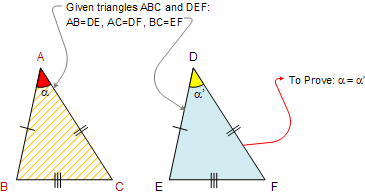Euclid's Elements Book I, Proposition 8: (Side-Side-Side SSS Congruence)
If two triangles have the two sides equal to two sides respectively, and have also the base equal to the base, they will also have the angles equal which are contained by the equal straight lines.

Let ABC and DEF be two triangles having the two sides AB and AC equal to the two sides DE and DF respectively, namely AB to DE, and AC to DF; and let them have the base BC equal to the base EF; I say that the angle BAC is also equal to the angle EDF.The Elements: Books I-XIII
 Euclid's Elements is the oldest mathematical and geometric treatise consisting of 13 books written by Euclid in Alexandria c. 300 BC. It is a collection of definitions, postulates, axioms, 467 propositions (theorems and constructions), and mathematical proofs of the propositions.